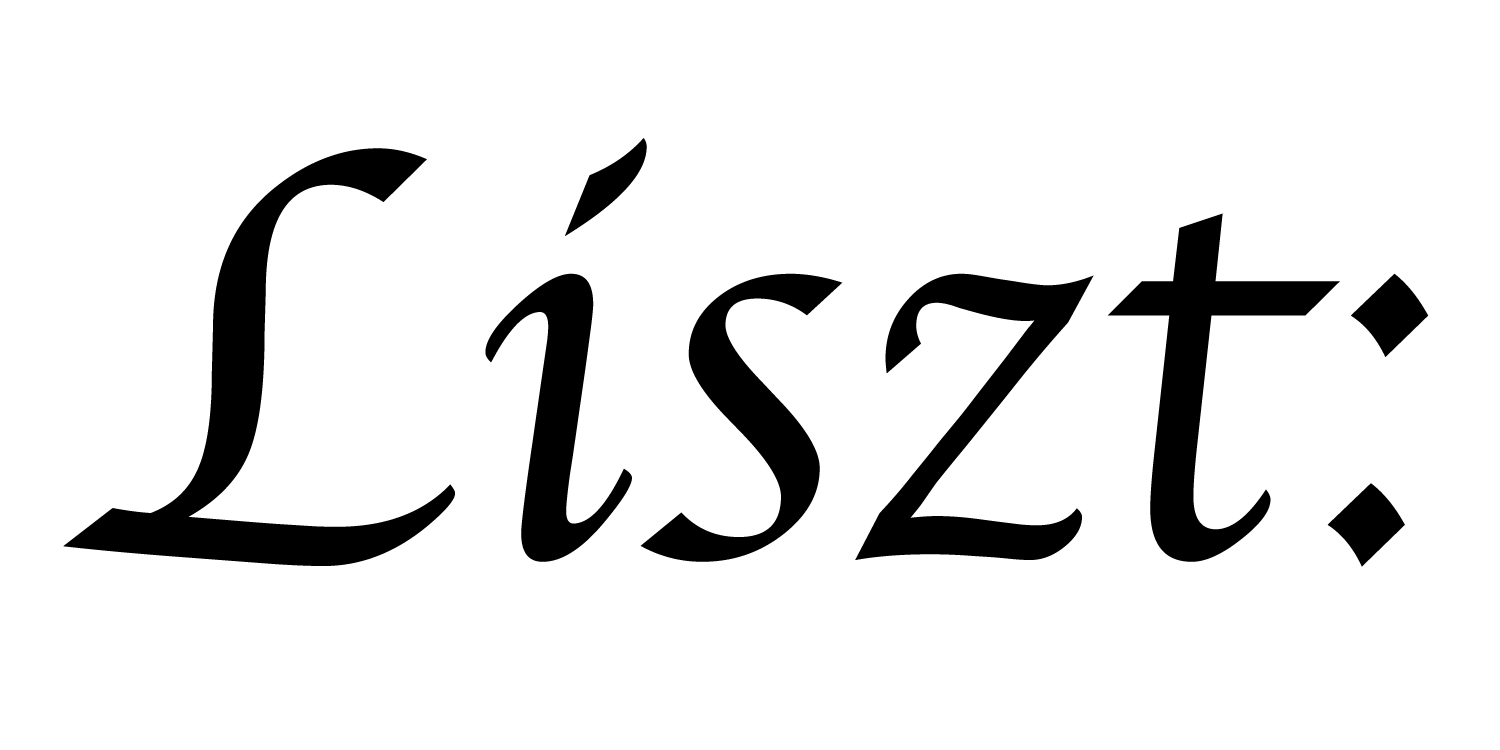# 15: Macros

In the last two tutorials, we saw a useful feature and trick which allows us to model arbitrary connectivity patterns between relations. However, these mechanisms require programmers to use an unintuitive interface: `L.Where(...)` loops. In earlier tutorials, we saw a much more intuitive loop syntax for triangle meshes: `v.edges`. The missing ingredient is a macro. In this tutorial, we explain how geometric domain authors can encapsulate and hide relational details behind more intuitive syntax.

Besides macros, we’ll also introduce field-functions. These two tools allow simulation and geometric domain authors to abstract functionality and retrofit old code.

Unlike previous tutorials, this file will not compute much, though it can still be safely executed.

``````import 'ebb'
local L = require 'ebblib'

local ioOff = require 'ebb.domains.ioOff'
local PN    = require 'ebb.lib.pathname'
local mesh  = ioOff.LoadTrimesh( PN.scriptdir() .. 'octa.off' )

local vdb   = require('ebb.lib.vdb')

local v_triples       = mesh.triangles.v:Dump({})
local tri_ids, v_ids  = {}, {}
for k=0,mesh.triangles:Size()-1 do
tri_ids[ 3*k + 1 ] = k
tri_ids[ 3*k + 2 ] = k
tri_ids[ 3*k + 3 ] = k
local triple = v_triples[k+1]
v_ids[ 3*k + 1 ] = triple
v_ids[ 3*k + 2 ] = triple
v_ids[ 3*k + 3 ] = triple
end

local triangles_of_vertex = L.NewRelation {
name = "triangles_of_vertex",
size = #tri_ids,
}

triangles_of_vertex:GroupBy('v')
``````

The program starts the same way as in the last tutorial; by defining a join-table.

``````local swap_macro = L.Macro(function(a, b)
return ebb quote
var tmp = a
a = b
b = tmp
in 0 end
end)
local ebb use_swap( v : mesh.vertices )
var a : L.int = 1
var b : L.int = 2
swap_macro(a, b)
L.print(b)
L.assert(b == 1)
end
mesh.vertices:foreach(use_swap)
``````

To start, we define a macro that swaps two values. This macro is defined by a Lua function that runs at compile time, returning a quoted piece of Ebb code. This quoted bit of code gets spliced into the ebb function below where swap_macro is called. That is, the macro gets substituted, rather than executed like a function. The design here is very similar to Terra, though less fully developed. (Note that the `in 0` is needed in case an Ebb quote is used somewhere where an expression is expected.)

Why not just define swap with another Ebb function? If we did that, then the two arguments to swap would be passed by value. Swapping them would accomplish nothing in the calling context. However, because a macro is substituted, the parameters are really just other bits of code containing the variable symbols/names. In general, macros are needed in some weird cases like these where we want to break the rules of normal function calls.

``````local triangle_macro = L.Macro(function(v)
return ebb `L.Where(triangles_of_vertex.v, v).tri
end)
mesh.vertices:NewFieldMacro('triangles', triangle_macro)
``````

One of the special features of Ebb is the ability to install macros on relations as if they were fields. Now that we’ve installed this macro, we can clean up the code for computing the dual-area of a vertex.

``````mesh.vertices:NewField('dual_area', L.double):Load(0.0)

local ebb compute_area ( t : mesh.triangles )
var e01 = t.v.pos - t.v.pos
var e02 = t.v.pos - t.v.pos

t.area = L.length( L.cross(e01, e02) )
end
mesh.triangles:foreach(compute_area)

local ebb compute_dual_area ( v : mesh.vertices )
for t in v.triangles do
v.dual_area += t.area
end
v.dual_area = v.dual_area / 3.0
end
mesh.vertices:foreach(compute_dual_area)
``````

Notice that the query loop in `compute_dual_area()` now reads `for t in v.triangles do` rather than `for t in L.Where(...).tri do`. Even though `L.Where(...)` is not a value that could be returned from a function, we can use a macro to abstract the snippet of code. By further using the `NewFieldMacro()` feature, we can make the user-syntax clean and uniform. This is how `v.edges` is defined in the standard triangle library.

``````mesh.vertices:NewField('density', L.double):Load(1.0)